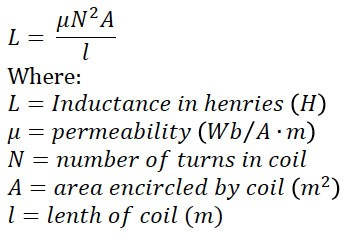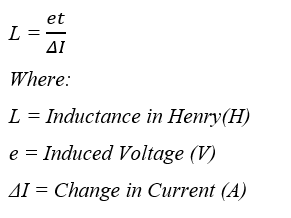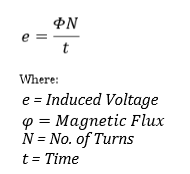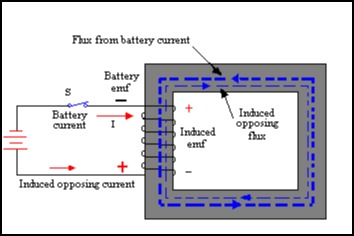# The Importance of Inductance of a Coil

In this blog we will explore Inductance; one of the major properties of an electrical circuit or an electronic device. Inductance is defined as a property of electrical circuit or a device that opposes a change in current. It is important to note that inductance does not oppose the current but rather opposes the change in current flowing within the circuit.

The unit of inductance is henry (H) and it is dependent on the physical properties of the circuit and not on the electrical attributes as there is no current or voltage involved. Additionally, Inductance is directly proportional to ‘Number of Turns’, ‘Area encircled by coil’ and inversely proportional to the ‘Length of coil’.

The symbol used to represent inductance is the capital letter L. The inductance (or more precisely, the self-inductance) of the coil can be found using the following equation:Applied Formula in Electrical Circuits:## Review of the Inductance Process

To understand the inductance process and how it works let’s review the illustrated DC supply with a switch and a coil (figure 1). When the switch is closed, current flows through the circuit. Here we use electron flow shown in red arrows as the direction of the electrons. When we close the switch, current flowing from the battery increases within each coil. Considering the Left-Hand Thumb Rule, the current flows away and the magnetic flux flows in the counterclockwise direction and expanding outwards. Taking into account Coil 1 and Coil 2 as in the figure; flux from Coil 1 is sweeping out to cross over Coil 2 creating an induced voltage. Lenz’s Law and Faraday’s Law are the two laws applied to understand this process. The induced voltage will always set up a flux that opposes the original flux that caused it. Thereby our applied voltage is trying to drive current into the coil and the induced voltage is working against that. It doesn’t stop the current, it just slows the current down. If Ohm’s Law dictated that the current would be 10 amps it won’t go from 0-10 instantly, instead it would take some time depending on the inductance provided by the inductor.

In the next case we will try to decrease the current, in the same circuit with 10 Amps as established, the magnetic flux is interlinking all the turns in the coil. When we open the switch, the flux retreats back to the original conductor. The movement of flux is stopped as the flow of current stopped changing. The decreasing magnetic flux over each conductor coil turn causes relative motion between the magnetic fields resulting in a highly induced voltage. The action to reduce the current/voltage to zero by removing the applied voltage leads the continuous change in magnetic flux that induces the voltage trying to oppose the cause. Thus the circuit tries to keep the current flowing leading to a delay in current reduction rate rather than reducing it instantly. This is referred to as Inductive Kick. The process taking place is simply the returning energy back to the circuit from the magnetic fields. The amount of voltage that is induced depends on factors such as amount of flux, number of turns and time.

The opening of a circuit can cause a very high induced voltage in the coil as the time is very short and the flux collapses very quickly. If there is no path for this energy to pass through, we can get arcs across the switches or arc close to other connected devices which can cause damages. So, some precautions are highly recommended when coming across the characteristics of inductance in electronic circuits.## Preventive Measures to Avoid Arc Flash

The inductive current produced in a DC circuit has a high strength when the flux related to the coil drops out instantaneously. It is therefore important to take preventive measures to eliminate the damage by providing an alternative path for the energy. For example, a current transformer is a highly inductive device as it has many turns of coil and the number of turns of the coil has a great impact on the current generated ( L = µN2A/l , where N = no. of turns ).Figure 2: Principle of Counter EMF

If the Ammeter attached with the coil as in Figure 2, is removed while the circuit is hot, the highly inductive circuit is interrupted. The opening of the circuit leads to the flux collapsing over the turns of coil producing an extremely high induced voltage that can draw an electric arc of 4-5 inches long. This electric arc can cause injury to the person trying to remove the ammeter from the circuit.

This is similar to the shunt winding of a DC motor, which is another highly inductive circuit. The DC shunt motor also satisfies all characteristics that generates a highly inductive current. The shunt motor is normally kept energized and modifications are made to another part of the motor to provide an alternative path for the inductive current to flow. This is done so as the switching of the shunt field can cause severe damages and shorten   the longevity of the contacts. Even in small coils used for PLC circuits, there is a kick to back-feed and do some damage.